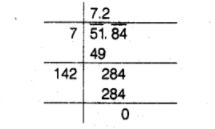# A decimal number is multiplied by itself.

Question:

A decimal number is multiplied by itself. If the product is 51.84, then find the number.

Solution:

Let the number be $x$. Then, product $=x \times x=x^{2}$

But product $=51.84$         [given]

$\therefore \quad x^{2}=51.84$

$\Rightarrow \quad x=\sqrt{51.84}$

Now, place the bar over the numbers, then square root is given below.

$\therefore \quad x=\sqrt{51.84}=7.2$Hence, the required number is $7.2$.